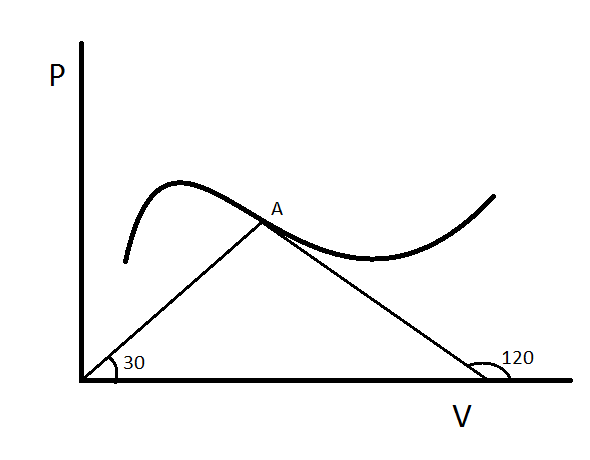# Molar Heat capacity$1$ Mole of an Ideal monoatomic gas undergoes a process depicted in the figure. At some point A of the $P-V$ diagram line joining the A to origin makes an angle $30$degrees with the $V$ axis and the tangent at point A makes an angle $120$ degrees with the $V$axis.

Find the instantaneous molar heat capacity of gas at point A in $cal mol^{-1} K^{-1}$ Take $R=2 cal mol^{-1} K^{-1}$

×

Problem Loading...

Note Loading...

Set Loading...